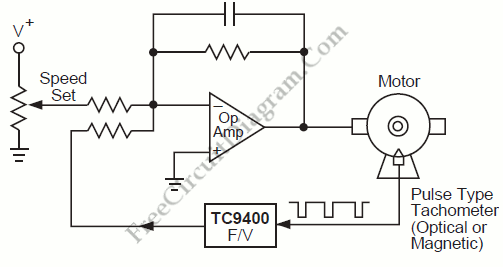# Simple Proportional-Integral Motor Speed ControlAnother example of frequency-to-voltage  converter application similar with RPM/Speed Indication is motor speed control. Here the converter is used to measure the actual speed which will be fed back to keep the actual speed stabilized according to a reference. Here is the block diagram of this control system:If the speed is to high, the output of the F/V converter will reduce the drive power delivered to the motor, and vice versa. The resistor across the output and the inverting input determine the proportional gain, and the capacitor determine the integral gain. This negative feedback mechanism is the core of this proportional motor speed control system. [System’s block diagram source: Microchip Application Note]